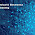## Search this site

1.(MATRIX CONCEPT), lecture n0- 5 is missing in MATLAB tutorial.

2.sir please upload 4,5,6,7,8 units of sdsd notes for eie 2nd yr

1.its very urgent

3.sir i have a slower network i cant download this much bigfile u can please tell me the website where i can buy this dvds

4.sir below is the list of video lectures mentioned as on youtube.
but i am not able to find lec 1 to lec 25. and lec 53,54,55.
Video Lectures on "VLSI Devices and Model" by Prof. S.K. Lahiri sir, IIT KGP
1. Semiconductor Materials and Structures
2. Semiconductor Band Structures
3. Electron and holes Statistics
4. Carrier Mobility and Conductivity
5. Carrier diffusion, generation/recombination, high-field effect
6. Avalanche Multiplication, Hall Effect
7. p-n junction formation, built-in potential
8. Quasi Fermi levels, p-n electrostatics
9. Abrupt and linearly graded p-n junction depletion layers and capacitance
10. Current - Voltage relation in p-n junction - I
11. Current - Voltage relation in p-n junction - II
12. Generation / recombination currents, diffusion capacitance
13. Diodes equivalent circuit, Breakdown voltage
14. Junction curvature effect, transient behaviour, noise
15. Tunnel diode, metal-semiconductor junctions
16. Schottky diodes, Ohmic contacts
17. Heterojunctions
18. Bipolar Junction Transistor, current-voltage relations
19. Current gain in BJT
20. Bandgap narrowing, Auger recombination, Early effect & punch-through in BJT
21. Breakdown voltage in BJT, small-signal equivalent circuit
22. Cut-off frequency of BJT, switching behaviour, HBT
23. HBT, SCR, JEFT
24. MOS devices classification, basic concepts of MOS field effect
25. Induced surface charge in MOS, accumulation, depletion & inversion layers
26. Capacitance-voltage characteristics, threshold voltage of MOS capacitor
27. Flat-Band voltage
28. Gate oxide charges, interface states, streching of CV plots
29. Transport through gate oxide, MOSFET
30. Current-voltage relation of long-channel MOSFETs
31. Drain conductance, transconductance, effect of drain-included channel depletion
32. Accurate model of drain current saturation, body effect
33. Drift-diffusion model, subthreshold conduction
34. Subthreshold slope, mobility model in MOSFETs
35. Temperature effect, eq circuit of MOSFET, threshold voltage control
36. Channel implantation, Substrate bias sensitivity
37. Scaling of MOSFETs, Short-channel effects
38. Charge sharing model
39. Narrow width effect, channel length modulation, hot carrier effects
40. MOSFET fabrication, self-aligned silicide technology., LDD MOSFET
41. VMOS, FAMOS, EEPROM
42. Oxide isolate CMOS, control of latch-up, SOI
43. Charge Coupled Devices (CCD)
44. MESFET, Permeable base transistor
45. Advanced high-speed devices, MODFET, Quantum devices
46. Level 1 model of MOSFET - I
47. Level 1 model of MOSFET - II
48. Level 2 model of MOSFET - I ; mobility modelling, subthreshold current, channel length modulation
49. Level 2 model of MOSFET - II ; short channel effect, velocity saturation
50. Level 2 model of MOSFET - III ; narrow width effect, gate capacitance
51. Level 2 model of MOSFET - IV ; junction capacitances
52. Level 3 model of MOSFET ; slope discontinuity, gate capacitances, BSIM model
53. BJT models : Basic Ebers-Moll model, basic Gummel-Poon model
54. Model derivation
55. Moll-Ross equation, high injection effect